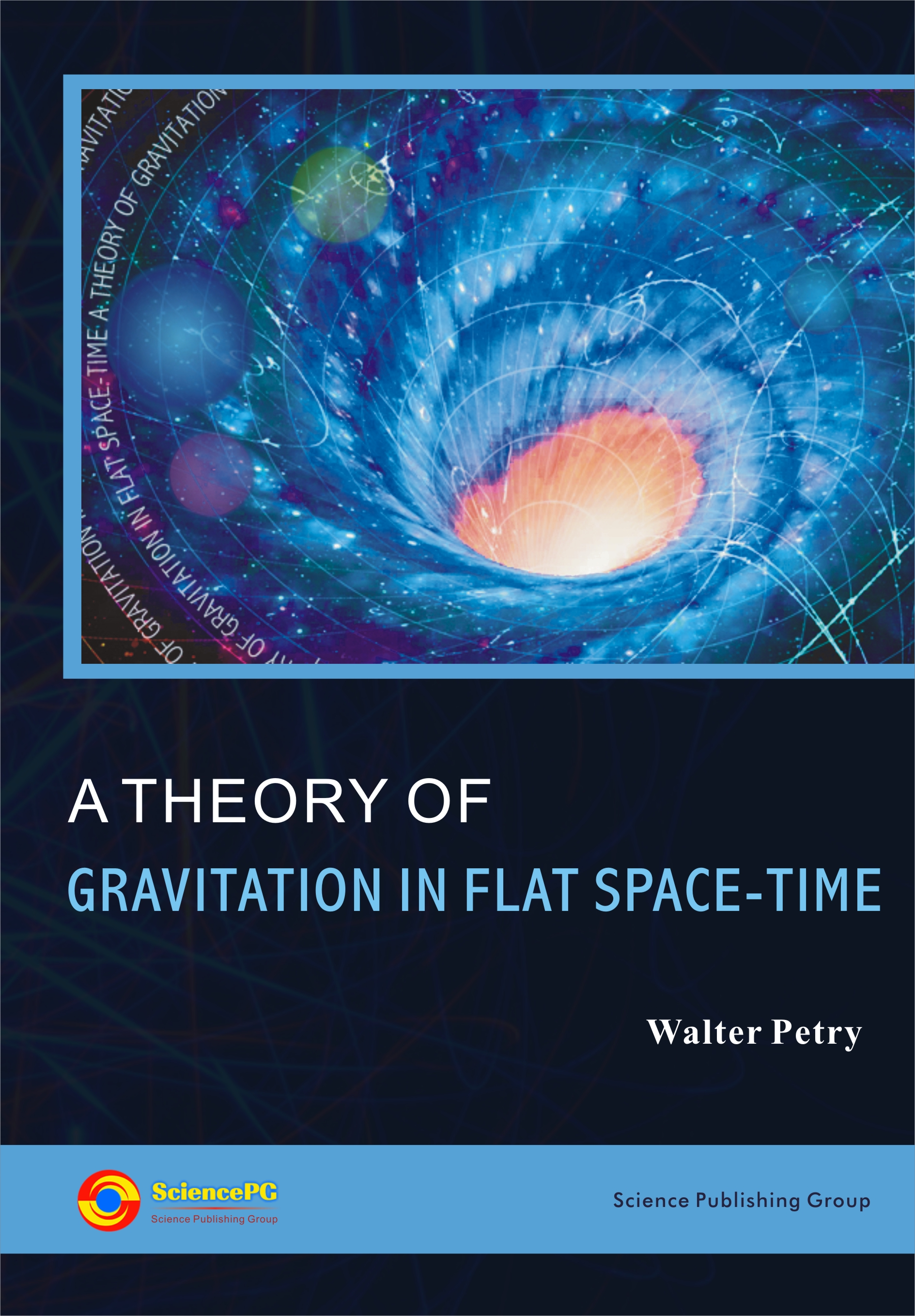Closed ×
Home / Books / Published Books / A Theory of Gravitation in Flat Space-TimeA Theory of Gravitation in Flat Space-Time
Author:
Walter Petry
ISBN:
978-1-940366-11-1
Published Date:
April, 2014
Pages:
182
Paperback:
\$89
Publisher:
Science Publishing GroupOPEN ACCESSThe Whole Book
Since May 20, 2015
Front Matter
Since September 19, 2014
Chapter 1 Theory of Gravitation
Since September 19, 2014
1.1 Gravitational Potentials
1.2 Lagrangian
1.3 Field Equations
1.4 Equations of Motion and the Energy-Momentum
1.5 Field Equations Rewritten
1.6 Field Strength and Field Equations
1.7 Angular-Momentum
1.8 Equations of the Spin Angular Momentum
1.9 Transformation to Co-Moving Frame
1.10 Approximate Solution in Empty Space
Chapter 2 Static Spherical Symmetry
Since September 19, 2014
2.1 Field Equations, Equations of Motion and Energy-Momentum
2.2 Gravitational and Inertial Mass
2.3 Gravitational Field in the Exterior
2.4 Non-Singular Solutions
2.5 Equations of Motion
2.6 Redshift
2.7 Deflection of Light
2.8 Perihelion Shift
2.10 Neutron Stars
Chapter 3 Non-Static Spherically Symmetry
Since September 19, 2014
3.1 The Field Equations
3.2 Equations of Motion and Energy-Momentum Conservation
Chapter 4 Rotating Stars
Since September 19, 2014
4.1 Field Equations
4.2 Equations of Motion
4.3 Rotating Neutron Stars
Chapter 5 Post-Newtonian Approximation
Since September 19, 2014
5.1 Post-Newtonian Approximation
5.2 Conservation Laws
5.3 Equations of Motion
Chapter 6 Post-Newtonian of Spherical Symmetry
Since September 19, 2014
6.1 Post-Newtonian Approximation of Non-Stationary Stars
6.2 2-Post-Newtonian Approximation of a Non-Stationary Star
6.3 Non-Stationary Star and the Trajectory of a Circulating Body
6.4 Gravitational Radiation from a Binary System
Chapter 7 The Universe
Since September 19, 2014
7.1 Homogeneous Isotropic Cosmological Models with Cosmological Constant
7.2 Homogeneous Isotropic Cosmological Model without Cosmological Constant
Chapter 8 Expanding or Non-Expanding Universe
Since September 19, 2014
8.1 Non-Expanding Universe
8.2 Proper Time and Absolute Time
8.3 Redshift
8.4 Age of the Universe
Chapter 9 Perturbations in the Universe
Since September 19, 2014
9.1 Differential Equations of Linear Perturbation in the Universe
9.2 Spherically Symmetric Perturbations
9.3 Beginning of the Universe
9.4 Matter dominated Universe
Chapter 10 Post-Newtonian Approximation in the Universe
Since September 19, 2014
10.1 Post-Newtonian Approximation
10.2 Equations of Motion
10.3 Newtonian and Long-Field Forces
Chapter 11 Preferred and Non-Preferred Reference Frames
Since September 19, 2014
11.1 Preferred Reference Frame
11.2 Non-Preferred Reference Frame
Chapter 12 Further Results
Since September 19, 2014
12.1 Anomalous Flyby
12.2 Equations of Maxwell in a Medium
12.3 Cosmological Models and the Equations of Maxwell in a Medium
12.4 Redshift of Distant Objects in a Medium
12.5 Flat Rotation Curves in Galaxies with Media
Back Matter
Since September 19, 2014
Author(s)
Walter Petry, Mathematisches Institut der Universitaet Duesseldorf, Duesseldorf, Germany
Description
The theory of gravitation uses a flat space-time metric, e.g., the pseudo-Euclidean geometry. The gravitational field is described by a tensor of order 2 (potentials) which satisfies covariant (relative to the metric) differential equations of order 2. The left hand side of the differential equations is the total energy-momentum tensor of matter and the gravitational field. The theory is a field theory in analogy to the equations of Maxwell for Electrodynamics and not a geometrical description of gravitation. Applications: The results of the theory agree for weak gravitational field with those of general relativity to the measurable order but for strong gravitational fields are differences, e.g. in the beginning of the universe. The space is flat by the theory. There are non-singular cosmological models, i.e., there is no big bang but the universe may be very hot in the beginning. The universe contracts to a small positive value and then it expands for all times. A new interpretation of a non-expanding universe can also be given. A modified Hubble law is also received in agreement with the results of Arp who states that there are in the universe nearby quasars with quite different redshifts. In addition, post- Newtonian approximations in the matter dominated universe can give large density contrasts. This is important to explain the large scale-structures in the universe, e.g. galaxies, etc. We need no inflation because the space is flat and not curved.
PUBLICATION SERVICES